# Simply Scheme Chapter 8 – Higher Order Functions

A function that takes another function as one of its arguments is called a higher-order function. This chapter discusses several such functions.

## Every

`every` is a function that lets us take a function as an argument and apply it to every word in a sentence or every letter in a word, regardless of the length of the sentence or word.

E.g. this gives you the first letter of every word in a sentence:

`every` is taking `first` as an argument, not invoking it.
`every` always returns a sentence.

## Keep

`keep` takes a predicate and sentence as arguments and returns the words for which the predicate is true. If given a word instead of a sentence, it returns the letters (in word form) for which the predicate is true.

## Accumulate

`accumulate` takes a procedure and sentence as arguments, applies that procedure to two elements of the sentence and gets a result. It then takes that result and applies the procedure to that result and the next element in the sentence, and keeps going until it’s combined everything together.

`accumulate` can also take a word as an argument instead of a sentence, treating the letters as elements.

## Combining Higher Order Functions

You can combine higher order functions e.g.:

The `keep number?` takes the argument `sent` and returns a new sentence where `number?` is true for each element. Then that sentence gets passed to `accumulate +`. So the `keep number?` essentially acts as a filter for non-numbers, keeping them out of `accumulate +`‘s way and insuring that the sentence argument for `accumulate +` only has numbers to deal with.

## Repeated

the `repeated` procedure returns a procedure that invokes the original procedure repeatedly. E.g.

`(repeated bf 3)` returns a procedure that does `(bf (bf (bf)))` on some input. If you don’t give it an input then Scheme just tells you that you have a procedure: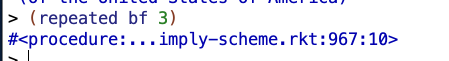## Shorter Notes

Scheme’s treatment of sentences as first-class data and functions as first-class lets us generalize ideas like “apply this function to every word of a sentence.

`keep` always returns a function of the same type as its second argument.

`every` always returns a sentence. If you want a word for `every`, you can `accumulate word` the sentence that `every` returns.

`every` expects a function of just one argument as its first argument. if you give it a function like `quotient` that expects two arguments, you’ll get an error message about not having enough arguments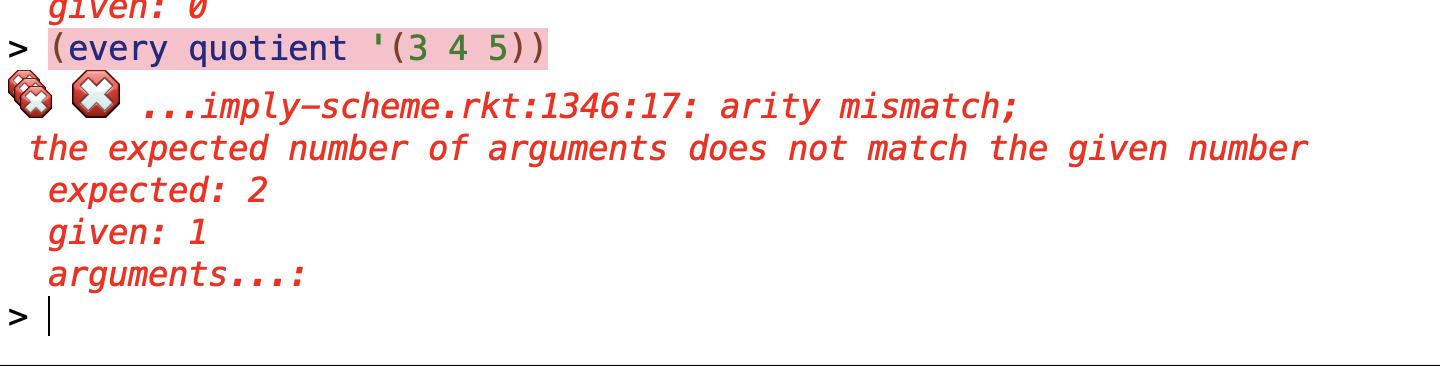Trying `(every (quotient 6) '(1 2 3))` doesn’t help. Scheme tries to evaluate `quotient 6` and throws an error.

This doesn’t work either: `(every (+ 3) '(1 2 3))`

`(+ 3)` returns 3. `every` wants a procedure as its first argument, and the number 3 isn’t a procedure: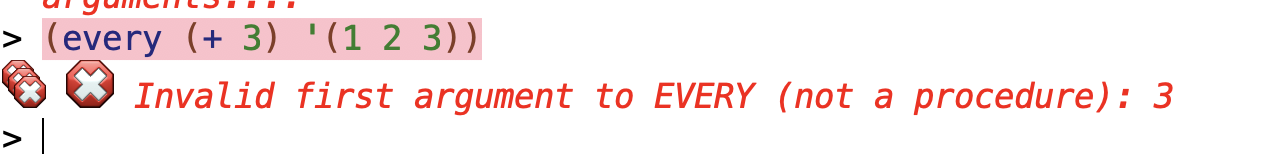`accumulate` can accept an empty sentence or word for `+`, `*`, `word`, and `sentence`.

## Boring Exercises

What does Scheme return as the value of each of the following expressions? Figure it out for yourself before you try it on the computer.

### ✅❌ Exercise 8.1

`(every last '(algebra purple spaghetti tomato gnu))`

I predicted: `'(aeiou)`
❌ Woops. It should be `(a e i o u)`

`(keep number? '(one two three four))`

I predicted `()`.
✅I was correct.

`(accumulate * '(6 7 13 0 9 42 17))`

I predicted 0.
✅ I was correct.

`(member? 'h (keep vowel? '(t h r o a t)))`

I predicted false. `keep vowel?` returns a sentence of `(o a)` and ‘h is not a member of that sentence.

✅ I was correct.

(every square (keep even? ‘(87 4 7 12 0 5)))

I predicted `'(16 144 0)`.

✅ I was correct.

(accumulate word (keep vowel? (every first ‘(and i love her))))

I predicted `'ai`.
✅ I was correct.

((repeated square 0) 25)

It just returns `25`. I guess repeated gets called 0 times? So you just return the value of the number. It’s interesting though … if you call `(repeated square 0)` you do get a procedure back. I wonder what the procedure is exactly.

(every (repeated bl 2) ‘(good day sunshine))

I predicted `'(go d sunshi)`.

✅ I was correct.

### ✅ Exercise 8.2

Fill in the blanks in the following Scheme interactions:

Forgot to include this one initially, didn’t see it until I saw Anne’s answer:

### ✅❌ Exercise 8.3

Describe each of the following functions in English. Make sure to include a description of the domain and range of each function. Be as precise as possible; for example, “the argument must be a function of one numeric argument” is better than “the argument must be a function.”

❌ (wrong) f takes a word or sentence consisting entirely of numbers as an argument.

If provided a word as the second argument, it will return the subset of the word that consists of even numbers.

If provided a sentence as the second argument, it will return the subset of words in the sentence that consist of even numbers.

`f`‘s domain is words or sentences made entirely of numbers.

Its range is a word or sentence that consists entirely of even numbers.

✅ (FIXED) The domain is non-negative integers (by themselves) or integers (including negative integers) in a sentence. Its range is non-negative even integers (by themselves) or even integers (including negative integers) in a sentence.

`g` takes a function that operates on one word. it applies that function to every element of the sentence `(blue jay way)` and returns a sentence with the result. e.g.:

❌ (WRONG) `g`‘s domain is functions that accept one word-arguments, and its range is a sentence that is some transformation of `(blue jay way)`.

✅ (CORRECTION) AnneB pointed out that you can actually get any output using `g` if you define a function that is always true and returns the sentence you want:

So the range is all sentences.

`h` takes a procedure `c` and calls it twice on an argument `d`.

For this procedure to work, the procedure used as the argument has to accept what it returns as input. So for example, `even?` would not work as a procedure because that returns booleans but does not accept them. `butfirst`, by contrast, both returns and accepts sentences, so that could work.

`h` can accept procedures that operate on numbers. for example:

So the domain for the procedure part (`c`) is a procedure that returns and can accept the same data type. There appear to be no restrictions on the domain in terms of the data part (`d`). I could not think of any restrictions on the range either.

`i` takes a number or sentence consisting of numbers and divides the total of the numbers by the number of numbers in the sentence (in other words, it finds the average)

❌ (WRONG)`i`‘s domain is a number or sentence consisting of numbers.
`i`‘s range is numbers.

✅ (FIXED) The domain is limited to positive integers or 0, or sentences with any numbers (including complex numbers and weird stuff like that). No empty words/sentences though (you get a division by 0 error)

`accumulate` takes a procedure that combines two arguments as its first argument, and a word or sentence as its second arguments, and goes through and combines the elements in the second argument until it runs out.

`accumulate` returns a combined value depending on the procedure provided to it.

for `word`, it takes a sentence and returns a combined word.
for `se`,
for `+`, it takes as sentence of numbers and returns the sum of those numbers.
for `-`, it seems to work a differently than you might expect. Consider `(accumulate - '(10 25 43 69 1 2))`. You might expect this procedure to subtract 25 from 10, getting -15, and then subtract 43 from -15, getting -58, and so on until arriving at a value of -130.

but

Why -42?

I think how the functions work (paralleling an example from the text) is something like this:

for accumulating a word, the order does not matter:

but for accumulating subtraction the order matters a lot.

Accumulate has a complicated domain and I’m not sure how to describe it comprehensively. Depending on the procedure provided, accumulate’s domain can include a word or sentence or number. For `+`, `*`, `word`, and `sentence`, accumulate can accept empty arguments.

Its range includes numbers, words or sentences.

AnneB says:

accumulate takes two arguments, a procedure and a word or sentence. The domain of accumulate is all sets of a procedure and a word or sentence that fit at least one of the following:

1. A procedure that can accept two letters (words of length one) or two words (whichever it is paired with) and returns something that it will accept as an argument, along with a word or sentence that is at least 2 in length.
2. Any procedure, along with a word or sentence of length 1.
3. The procedures +, *, word, or sentence, along with an empty word or an empty sentence.

I’m not sure 2 is correct. Certain procedures don’t take certain inputs (like even? doesn’t take words) and will give error if you try to use them.
1 and 3 seem correct. More AnneB:

accumulate calls the procedure with the first two elements of the given word or sentence, then calls it again with that result and the third element, and continues until it has used all the elements of the given word or sentence. If the given word or sentence has only one element, accumulate returns that word or sentence. If the given word or sentence is empty, accumulate returns the identity element of the given procedure.

I agree.

`sqrt` takes a number and returns the square root of that number.
`sqrt`‘s domain is numbers and its range is a real or imaginary number.

This may be wrong re: range. I think maybe I meant complex numbers and not imaginary numbers. Anne wrote a lot of stuff about it:

The domain of sqrt is at least all real numbers. The version of Scheme I’m using does accept negative numbers. For instance:

`> (sqrt -1)`
`0+1i`

I can’t figure out if there’s a way to input complex numbers like “2+3i” into this version of Scheme.

If you enter them as number + i, it works: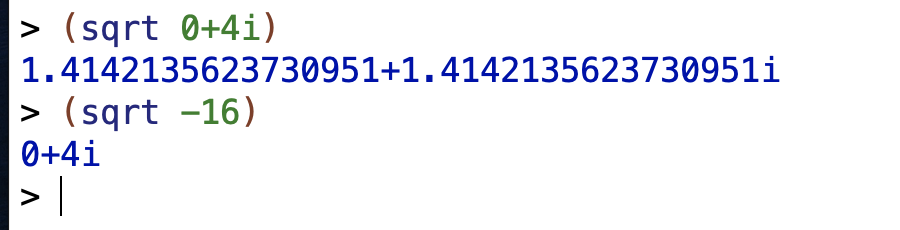Just `4i` by itself won’t work though. It’s detecting only the specific number+i format, at least for me.

If there is, then the domain might include them too.

Ya it seems to include complex numbers.

I found this:

Mathematically, numbers may be arranged into a tower of subtypes in which each level is a subset of the level above it:
number
complex
real
rational
integer
For example, 3 is an integer. Therefore 3 is also a rational, a real, and a complex. The same is true of the Scheme numbers that model 3. For Scheme numbers, these types are defined by the predicates number?, complex?, real?, rational?, and integer?.

The sqrt procedure returns the principal square root of the number. This place explains what that is:

procedure: sqrt z
Returns the principal square root of z. The result will have either positive real part, or zero real part and non-negative imaginary part.

So if the domain of sqrt is the real numbers, then the range is the non-negative real numbers plus the complex numbers with a zero real part and non-negative imaginary part. If the domain of sqrt is the complex numbers, I’m not sure what the range is, but it might be all complex numbers with a positive real part or a zero real part and a non-negative imaginary part. If the domain of sqrt is all numbers, then I don’t know what the range is.

Seems fair to say `sqrt`‘s domain is complex numbers and range is complex numbers with a positive real part or a zero real part and a non-negative imaginary part.

`repeated` takes a procedure and a number and returns a procedure which consists of the procedure it was provided invoked a number of times equal to the number it was provided. e.g.:
`((repeated bf 3)'(potato cheese ninja puff hat))`
returns `'(puff hat)`.

`repeated`‘s domain is a procedure and positive integer or 0, and its range includes many procedures. If the number provided to `repeated` is greater than 1, the procedure has to be able to take the value it returns as an input. For example, `((repeated even? 1) 2)` works because `even?` is only getting invoked one time. OTOH, `((repeated even? 2) 2)` does not work because the first invocation of `even?` returns a boolean, and `even?` returns but does not take booleans.

Forgot to discuss giving 0 as the second argument. If you use 0 as the number of times to invoke the procedure, then you just get the word or sentence returned back: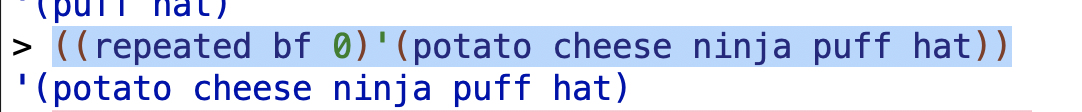AnneB says:

repeated takes two arguments, a procedure and a number. The domain of repeated is all sets of procedures and whole numbers where the range of the procedure is a subset of the domain of the procedure (it doesn’t have to be a proper subset),

I think maybe “all sets of procedures and whole numbers where the range of the procedure is a subset of the domain of the procedure” is trying to get at a similar point as I was trying to get at with “If the number provided to `repeated` is greater than 1, the procedure has to be able to take the value it returns as an input.” The range has to be a subset of the domain so that the procedure can be called repeatedly in cases where you invoke the procedure > 1 times.

or the number is 0 or 1 and the procedure is any procedure. The range of repeated is the union of all the ranges of such procedures.

I think maybe I follow the part re: “union” but I’m not really sure. I think maybe it means that the range of repeated is the range of all the procedures previously described combined.

`(repeated sqrt 3)` takes a number and finds its square root 3 times. For example, `(repeated sqrt 3)` applied to 256 returns 2. (The square root of 256 is 16, the square root of 16 is 4, the square root of 4 is 2).

Seems like this would be the same as square root: domain is complex numbers and range is complex numbers with a positive real part or a zero real part and a non-negative imaginary part.

`(repeated even? 2)` returns a procedure which applies `even?` to a number, and then applies `even?` to the result of the first application of `even?`.

So in other words, it does something like:

`(even? (even? 2))`

If it was just `even?` once, then the domain would be a number and the range would be a boolean. With this function, however, things are different.

`even?` doesn’t accept booleans, which is all that `even?` can produce. You get an error message with this function regardless of the input.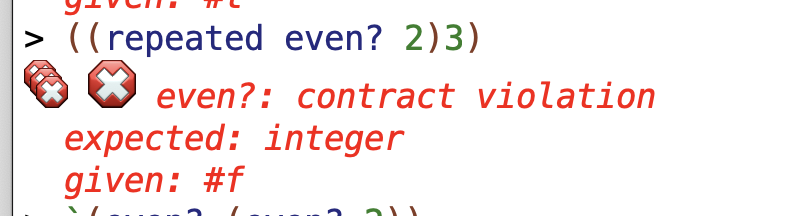So I think this function doesn’t have a domain or range. It’s like a broken procedure.

`(repeated first 2)` returns a procedure which takes takes `first` of some argument twice. So it does something like `(first (first '(potato tomato)))`.

Invoking `first` twice on a sentence first returns the first word of that sentence and then returns the first letter of the first word.

Invoking `first` twice on a word returns the first letter of that word. It doesn’t matter how many times you invoke `first` on a word repeatedly – you keeping getting the first letter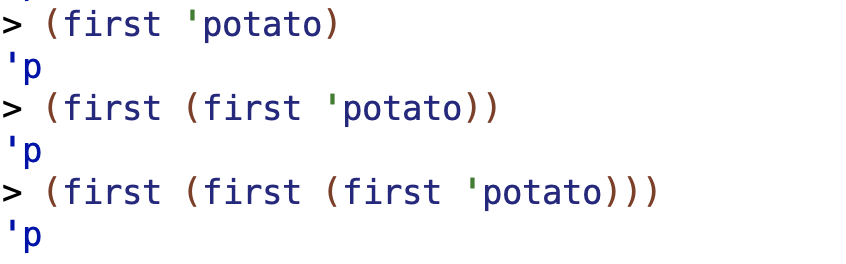So `(repeated first 2)` has the interesting property that it returns either the first letter of a word (or first character of a number) or the first letter of the first word of a sentence. For words and unnested sentences, it has the same output (a word consisting of a single letter).

If a sentence is nested one level, like `'((potato tomato))`, it will return the first word of the sentence (`potato` in my example).

If a sentence is nested two levels, like `'(((potato tomato)))`, the function will return the entire sentence. If there are multiple nested sentences, it will return the first sentence.

There are invalid inputs to `(repeated first 2)`. These include the empty sentence`'()` and empty word `""`.

❌ (wrong) The domain of `(repeated first 2)` is any non-empty word or sentence, and the range is either the first letter of a word or the first sentence within a nested sentence. Putting it more generally, we could say the range is the first element within a word or sentence (and keep in mind that with nesting the “first element” could be a whole nested sentence).

✅ (fixed). The domain of `(repeated first 2)` is any non-empty word or sentence. The range may likewise be non-empty words or sentences but I’m not sure.

`(repeated (repeated bf 3) 2)` results in `butfirst` being invoked 6 times.

the `(repeated bf 3)` returns a procedure that invokes `butfirst` 3 times. then the left-most `repeated` returns a procedure which invokes the `(repeated bf 3)` procedure twice on some input, resulting in 6 invocations.

We can see this in the following examples.

I defined the following function: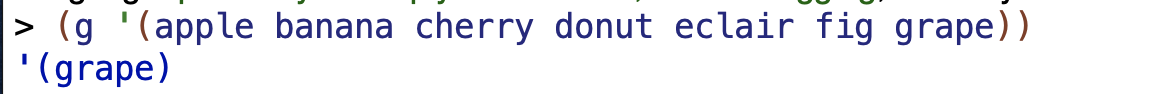`butfirst` 6 times on the input sentence returns the 7th value, grape.`butfirst` 6 times on the input returns a word starting at the 7th letter.

inputs to the function need to at least contain 6 values (in that case returning an empty list or sentence). If they have 5 or fewer values, an error will result.

So the domain of the function is any word or sentence with greater than 5 elements. The range is a word or sentence.

## Real Exercises

### ✅ Exercise 8.4

Write a procedure `choose-beatles` that takes a predicate function as its argument and returns a sentence of just those Beatles (John, Paul, George, and Ringo) that satisfy the predicate. For example:

### ✅ Exercise 8.5

Write a procedure transform-beatles that takes a procedure as an argument, applies it to each of the Beatles, and returns the results in a sentence:

### ✅ Exercise 8.6

When you’re talking to someone over a noisy radio connection, you sometimes have to spell out a word in order to get the other person to understand it. But names of letters aren’t that easy to understand either, so there’s a standard code in which each letter is represented by a particular word that starts with the letter. For example, instead of “B” you say “bravo.”
Write a procedure `words` that takes a word as its argument and returns a sentence of the names of the letters in the word:**

(You may make up your own names for the letters or look up the standard ones if you want.)
Hint: Start by writing a helper procedure that figures out the name for a single letter.

### ✅ Exercise 8.7

Write a procedure `letter-count` that takes a sentence as its argument and returns the total number of letters in the sentence:

### ✅ Exercise 8.8

Write an `exaggerate` procedure which exaggerates sentences:

It should double all the numbers in the sentence, and it should replace “good” with “great,” “bad” with “terrible,” and anything else you can think of.

### ✅ Exercise 8.9

What procedure can you use as the first argument to `every` so that for any sentence used as the second argument, `every` returns that sentence?

`sentence` seems to work, though for nested sentences it doesn’t return the nesting.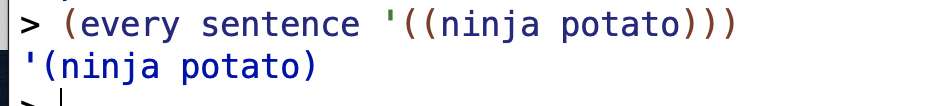AnneB noticed a couple of other things that work – `word` and a function that just returns the same input:

What procedure can you use as the first argument to `keep` so that for any sentence used as the second argument, `keep` returns that sentence?

`word?` seems to work for this, assuming no nesting. It works for the empty sentence too.

What procedure can you use as the first argument to `accumulate` so that for any sentence used as the second argument, `accumulate` returns that sentence?

`sentence` works, including for nesting.

### ✅ Exercise 8.10

Write a predicate `true-for-all?` that takes two arguments, a predicate procedure and a sentence. It should return `#t` if the predicate argument returns true for every word in the sentence.

### ✅ Exercise 8.11

Write a GPA procedure. It should take a sentence of grades as its argument and return the corresponding grade point average:

Hint: write a helper procedure `base-grade` that takes a grade as argument and returns 0, 1, 2, 3, or 4, and another helper procedure `grade-modifier` that returns −.33, 0, or .33, depending on whether the grade has a minus, a plus, or neither.*

Thought the slight implementation difference between my version and AnneB’s was interesting. AnneB added the modifiers up separately in the `gpa` part of the procedure while I added the modifier directly to the grade in `base-grade` using the `grade-modifier` helper procedure. I think maybe Anne’s organization is a bit more logical cuz it keeps the base grade and modifier separate until the final step.

### ✅ Exercise 8.12

When you teach a class, people will get distracted if you say “um” too many times. Write a `count-ums` that counts the number of times “um” appears in a sentence:

### ✅ Exercise 8.13

Write a procedure `phone-unspell` that takes a spelled version of a phone number, such as `POPCORN`, and returns the real phone number, in this case `7672676`. You will need to write a helper procedure that uses an 8-way `cond` expression to translate a single letter into a digit.

### ✅ Exercise 8.14

Write the procedure `subword` that takes three arguments: a word, a starting position number, and an ending position number. It should return the subword containing only the letters between the specified positions:

I should think more carefully about handling erroneous inputs with `else` clauses and that kind of thing.